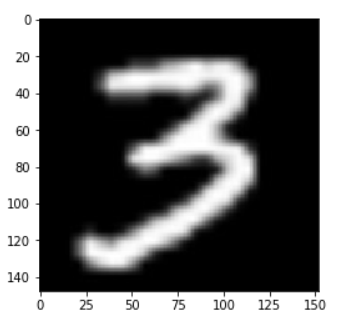# 训练模型

``````import numpy as np
from PIL import Image
import matplotlib.pyplot as plt
``````

``````# 定义多层感知器
def multilayer_perceptron(input):
# 第一个全连接层，激活函数为ReLU
hidden1 = fluid.layers.fc(input=input, size=100, act='relu')
# 第二个全连接层，激活函数为ReLU
hidden2 = fluid.layers.fc(input=hidden1, size=100, act='relu')
# 以softmax为激活函数的全连接输出层，大小为label大小
fc = fluid.layers.fc(input=hidden2, size=10, act='softmax')
return fc
``````

``````# 卷积神经网络
def convolutional_neural_network(input):
# 第一个卷积层，卷积核大小为3*3，一共有32个卷积核
conv1 = fluid.layers.conv2d(input=input,
num_filters=32,
filter_size=3,
stride=1)

# 第一个池化层，池化大小为2*2，步长为1，最大池化
pool1 = fluid.layers.pool2d(input=conv1,
pool_size=2,
pool_stride=1,
pool_type='max')

# 第二个卷积层，卷积核大小为3*3，一共有64个卷积核
conv2 = fluid.layers.conv2d(input=pool1,
num_filters=64,
filter_size=3,
stride=1)

# 第二个池化层，池化大小为2*2，步长为1，最大池化
pool2 = fluid.layers.pool2d(input=conv2,
pool_size=2,
pool_stride=1,
pool_type='max')

# 以softmax为激活函数的全连接输出层，大小为label大小
fc = fluid.layers.fc(input=pool2, size=10, act='softmax')
return fc
``````

``````# 定义输入层
image = fluid.layers.data(name='image', shape=[1, 28, 28], dtype='float32')
label = fluid.layers.data(name='label', shape=, dtype='int64')
``````

``````# 获取分类器
# model = multilayer_perceptron(image)
model = convolutional_neural_network(image)
``````

``````# 获取损失函数和准确率函数
cost = fluid.layers.cross_entropy(input=model, label=label)
avg_cost = fluid.layers.mean(cost)
acc = fluid.layers.accuracy(input=model, label=label)
``````

``````# 获取测试程序
test_program = fluid.default_main_program().clone(for_test=True)
``````

``````# 定义优化方法
opts = optimizer.minimize(avg_cost)
``````

``````# 获取MNIST数据
``````

``````# 定义一个使用CPU的执行器
place = fluid.CPUPlace()
exe = fluid.Executor(place)
# 进行参数初始化
exe.run(fluid.default_startup_program())
``````

``````# 定义输入数据维度
feeder = fluid.DataFeeder(place=place, feed_list=[image, label])
``````

``````# 开始训练和测试
for pass_id in range(5):
# 进行训练
train_cost, train_acc = exe.run(program=fluid.default_main_program(),
feed=feeder.feed(data),
fetch_list=[avg_cost, acc])
# 每100个batch打印一次信息
if batch_id % 100 == 0:
print('Pass:%d, Batch:%d, Cost:%0.5f, Accuracy:%0.5f' %
(pass_id, batch_id, train_cost, train_acc))

# 进行测试
test_accs = []
test_costs = []
test_cost, test_acc = exe.run(program=test_program,
feed=feeder.feed(data),
fetch_list=[avg_cost, acc])
test_accs.append(test_acc)
test_costs.append(test_cost)
# 求测试结果的平均值
test_cost = (sum(test_costs) / len(test_costs))
test_acc = (sum(test_accs) / len(test_accs))
print('Test:%d, Cost:%0.5f, Accuracy:%0.5f' % (pass_id, test_cost, test_acc))
``````

``````Pass:0, Batch:0, Cost:3.50138, Accuracy:0.07812
Pass:0, Batch:100, Cost:0.14832, Accuracy:0.96875
Pass:0, Batch:200, Cost:0.13408, Accuracy:0.96875
Pass:0, Batch:300, Cost:0.11601, Accuracy:0.97656
Pass:0, Batch:400, Cost:0.27977, Accuracy:0.92969
Test:0, Cost:0.08879, Accuracy:0.97379
Pass:1, Batch:0, Cost:0.11175, Accuracy:0.96875
Pass:1, Batch:100, Cost:0.07854, Accuracy:0.97656
Pass:1, Batch:200, Cost:0.04025, Accuracy:0.99219
Pass:1, Batch:300, Cost:0.09936, Accuracy:0.98438
Pass:1, Batch:400, Cost:0.19245, Accuracy:0.95312
Test:1, Cost:0.10123, Accuracy:0.97241
Pass:2, Batch:0, Cost:0.13749, Accuracy:0.96094
Pass:2, Batch:100, Cost:0.06074, Accuracy:0.98438
Pass:2, Batch:200, Cost:0.01982, Accuracy:0.99219
Pass:2, Batch:300, Cost:0.06725, Accuracy:0.97656
Pass:2, Batch:400, Cost:0.10043, Accuracy:0.96875
Test:2, Cost:0.13354, Accuracy:0.96776
Pass:3, Batch:0, Cost:0.08895, Accuracy:0.98438
Pass:3, Batch:100, Cost:0.06339, Accuracy:0.96875
Pass:3, Batch:200, Cost:0.05107, Accuracy:0.98438
Pass:3, Batch:300, Cost:0.08062, Accuracy:0.97656
Pass:3, Batch:400, Cost:0.07631, Accuracy:0.96875
Test:3, Cost:0.11465, Accuracy:0.97449
Pass:4, Batch:0, Cost:0.01259, Accuracy:1.00000
Pass:4, Batch:100, Cost:0.01203, Accuracy:1.00000
Pass:4, Batch:200, Cost:0.08451, Accuracy:0.97656
Pass:4, Batch:300, Cost:0.16532, Accuracy:0.98438
Pass:4, Batch:400, Cost:0.09657, Accuracy:0.98438
Test:4, Cost:0.14624, Accuracy:0.97211
``````

# 预测图像

``````# 对图片进行预处理
im = Image.open(file).convert('L')
im = im.resize((28, 28), Image.ANTIALIAS)
im = np.array(im).reshape(1, 1, 28, 28).astype(np.float32)
im = im / 255.0 * 2.0 - 1.0
return im
``````

``````!wget https://github.com/yeyupiaoling/LearnPaddle2/blob/master/note4/infer_3.png?raw=true -O 'infer_3.png'
``````

``````img = Image.open('infer_3.png')
plt.imshow(img)
plt.show()
````````````# 加载数据并开始预测
results = exe.run(program=test_program,
feed={'image': img, "label": np.array([]).astype("int64")},
fetch_list=[model])
``````

``````# 获取概率最大的label
lab = np.argsort(results)
print("该图片的预测结果的label为: %d" % lab[-1])
``````

``````该图片的预测结果的label为: 3
``````